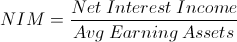Net Interest Margin

Net Interest Margin Calculator (Click Here or Scroll Down)Net interest margin is a formula used to evaluate how well a bank is using it's earning assets to produce a (net) interest income.

Net interest income, the numerator, is calculated by subtracting interest expenses from interest income. These are found on the bank's income statement. The denominator, average earnings assets, can be found in a company's 10-k, often specifically in the income statement or balance sheet. The net interest margin, itself, is also found on the bank's financial statements.

Not all income for banks is earned through interest income. Banks also charge fees for various services. This includes mortgage services, investment services, banking services, and card services. Banks also incur expenses unrelated to interest expenses. These expenses include equipment, payroll, and other similar operating expenses.

Nevertheless, interest income and expenses make up a large portion of a bank's income statement and the net interest margin is one metric used to gauge how well a bank is turning a profit for that period.

Example of Net Interest Margin

An example of net interest margin would be if a bank reports net interest margin of 2.67% on its 10-k form. This can be confirmed by looking at the company's net interest income of \$4 million and it's average earning assets of \$150 million. Although the information is directly provided, it is still important to understand how it is calculated. Perhaps there is a situation where an individual would like to investigate why last year's margin is higher than this year's margin. From here, by understanding and knowing the variables involved, this individual could look at where this change has occurred.

Use of Net Interest Margin

The net interest margin formula can be used internally by banks to gauge its profitability. It can also be used by investors looking to invest in a bank.

However, the net interest margin formula does not offer much in isolation. As stated earlier, banks have additional non-interest income and incur operating expenses. This particular formula would be part of a subset of formulas that are used collectively.

The net interest margin formula can also be used for an overall review of the banking industry, as well as the economy writ large.

The net interest margin for all US Banks can found by the Federal Reserve Bank of St. Louis here: https://fred.stlouisfed.org/series/USNIM

For countries outside the US, the Federal Reserve Bank of St Louis has additional metrics for some foreign countries. Also, each country's central bank will likely have metrics for that particular country.